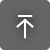# TL Clothoid

OS:

## 説明

An Euler spiral is a curve whose curvature changes linearly with its curve length (the curvature of a circular curve is equal to the reciprocal of the radius). Euler spirals are also commonly referred to as spiros, clothoids, or Cornu spirals.
Clothoids have applications to diffraction computations. They are also widely used as transition curves in railroad engineering/highway engineering for connecting and transitioning the geometry between a tangent and a circular curve. A similar application is also found in photonic integrated circuits. The principle of linear variation of the curvature of the transition curve between a tangent and a circular curve defines the geometry of the Euler spiral.

This app provides various commands allowing you to work with stand-alone spiral curves as polylines inside AutoCAD. Note that similar functionality exists out of the box in some Autodesk® AutoCAD® vertical products such as Autodesk® Civil 3D®. However:
- this app works in any AutoCAD based application, it does not require Civil 3D
- this app is designed not to replace, but to extend existing functionality found in Civil 3D, particularly while working with stand alone spirals

A Clothoid has various parameters (refer to the included diagram) such as:

• <Parameter A>: A scale value
• <Length>: The distance from sta 0 to the end of the curve. (Actual mathematical curve has a distance of +-Infinity and radius of zero at the end)
• <Radius>: The radius at the end of the computed curve at station +-<Length>
• <Tangent>: The value of the first derivative at the end of the curve at station +-<Length>. Range is 0 to Pi

Each set of two parameters uniquely defines a Clothoid for a total of six possible combinations. The app supports all combinations, each one implemented as a separate Clothoid creation command.

Point coordinates (x,y) along a normalized Clothoid curve are expressed by the Fresnel Integrals. These integrals don't have closed form solutions and are in turn approximated as power series. The app computes the first 21 terms of the Fresnel integral series approximations for each Clothoid point resulting in a sub millimeter accuracy for the initial few 'rounds' of the spiral (assuming the curve is computed in meters). However the initial curve computation is artificially limited to a tangent range of 0 to 2*Pi, which should suffice for all practical purposes. Note that additional polylines may be generated after the fact extending this initial range, utilizing the TLClothoidDrawSegment command (as far as the MaxError parameter value is reached)

MaxError setting: the app keeps track of the achieved accuracy internally for each computed pair of coordinated and rejects all points which exceed this max allowed value. The error (deviation from the true coordinates) is defined as the square root of the sum of the last three abs terms squared.
Error.X = Sum[Abs[(Term[i].X)],{i,19,21}]]
Error.Y = Sum[Abs[(Term[i].Y)],{i,19,21}]]
Error = Sqrt[(Error.X)^2 + (Error.Y)^2]

Features:

• Six clothoid creation commands
• Six additional clothoid manipulation/inquiry commands
• Dynamic preview of the computed curve
• Custom UCS support
• Error checking and accuracy control of the computed clothoid points
• Control of the number of polyline points/distance between computed points
• Parameters report for each computed curve
• XData with Clothoid definition parameters added to all generated polylines, use Express Tools to query the xdata

## このバージョンについて

バージョン 2022.1.20.1, 2022/02/28
Initial release

## カスタマ レビュー

0 件のレビュー
テクニカル ヘルプを表示